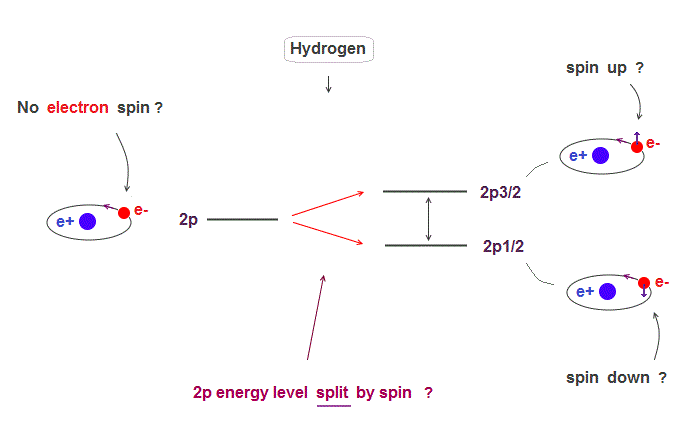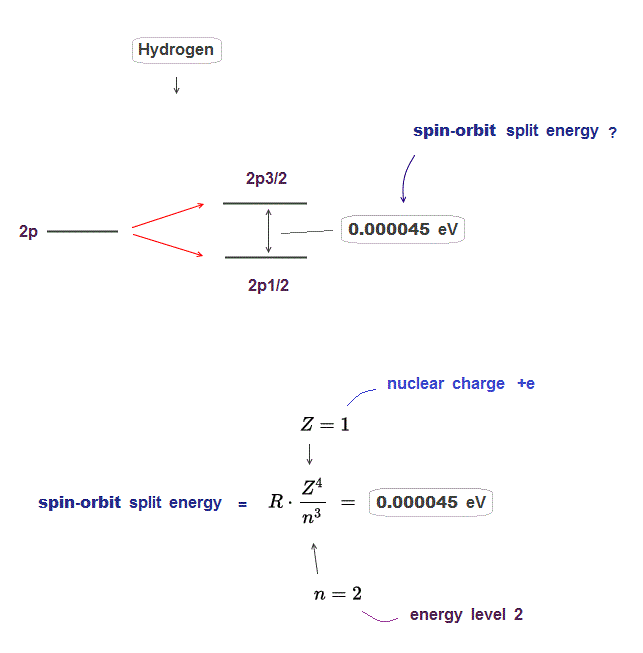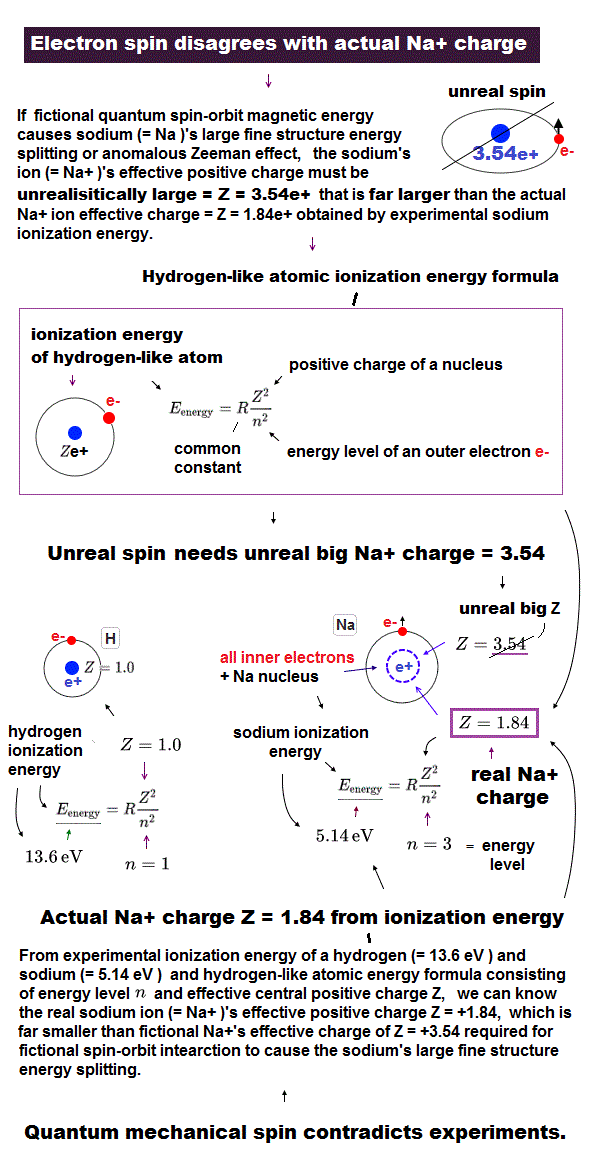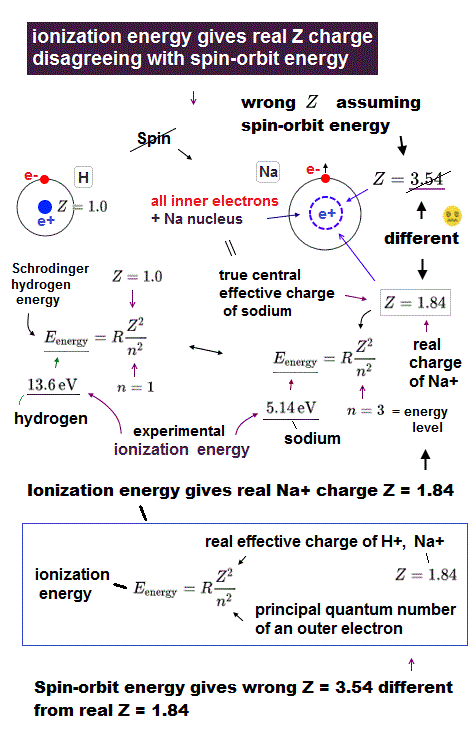﻿ Spin-orbit coupling is wrong and illusion

Spin - orbit coupling is illusion.

Hydrogen energy splitting is due to spin-orbit ?

[ Hydrogen 2p energy splits by spin-orbit interaction ? ]

(Fig.1)  Hydrogen fine structure (= 2p splits into 2p3/2 and 2p1/2 ) ?The small splitting of spectral line is seen in Hydrogen 2p energy level ( 2p → 2p3/2, 2p1/2 ).

Quantum mechanics claims this splitting is due to spin-orbit effect where electron spin is up ( down ) in 2p3/2 ( 2p1/2 ) with respect to its orbital angular momentum.

But electrons spin exceeding light speed is unrealistic.  This 2p splitting (= called fine structure ) can be explained by Bohr-Sommerfeld model without spin.

Hydrogen energy splitting is too small.

[ Hydrogen 2p energy splitting is only 0.000045 eV. ]

(Fig.2)  The enregy difference between 2p3/2 and 2p1/2 is only 0.000045 eV.Hydrogen 2p energy splitting is very small, the energy difference between 2p3/2 and 2p1/2 is only 0.000045 eV.

This energy difference is known to be expressed by the equation which is proportional to Z4/n3 ( Z is nuclear charge, n is principal quantum number ).

In 2p energy level in hydrogen, nuclear charge Z is 1, principal quantum number n is 2, angular momentum l is 1.

If you insert these numbers into the spin-orbit equation ( this p.4 ), you can get the above 0.000045 eV.

Sodium spin-orbit effect is too strong to believe !

[ Sodium spin-orbit effect is 100 times stronger than hydrogen ! ]

(Fig.3)  This Na large energy splitting is NOT due to spin-orbit !The problem is sodium 3p energy splitting is too large to explain by spin-orbit effect.

Sodium 3p electrons has n = 3, l = 1 and its energy splitting = 0.0021 eV, which is 100 times stronger than hydrogen (= 0.000045 eV ).

By inserting these numbers into the above spin-orbit equation, we obtain central positive charge Z = 3.5 which is much larger than hydrogen nuclear charge Z = 1.

This sodium central charge means Na+ ion (= including sodium nucleus and all other inner electrons ), so Z should be close to 1 like hydrogen.

So the sodium 3p energy splitting is NOT spin-orbit but strong Coulomb effect !

Spin-orbit theory fails in Alkali metals !

[ Energy splitting in alkali metals is too large for spin-orbit effect. ]

(Fig.4)  ↓ Sodium real central charge is Z = 1.4 ( not 3.5 ! ) by ionization energyThe real central positive charge Z can be estimated from sodium ionization energy of outer electron using hydrogen energy solution.

The energy required to ionize sodium outer 3p electron is 3.14 eV, which leads to central change Z = 1.4.

If we insert this "real" sodium central change Z = 1.4 into the above spin-orbit equation, we obtain very small 3p energy splitting (= only 0.000054 eV ).

It is far smaller than the experimental value (= 0.0021 eV ) indicating those energy splitting is NOT due to spin-orbit effect but due to Coulomb effect by sodium inner electrons.

In larger alkali atoms (= K, Rb, Cs .. ), these energy splitting is far larger, and it's more impossible to apply unreal spin-orbit effect to them !2017/1/20 updated. Feel free to link to this site.